How to Learn in 24 Hours?

 Need Help? M-F: 9am-5pm(PST): Toll-Free: (877) RAPID-10 or 1-877-727-4310 24/7 Online Technical Support: The Rapid Support Center vip@rapidlearningcenter.com Secure Online Order:Need Proof? Testimonials by Our Users

 Rapid Learning Courses: MCAT in 24 Hours (2021-22) USMLE in 24 Hours (Boards) Chemistry in 24 Hours Biology in 24 Hours Physics in 24 Hours Mathematics in 24 Hours Psychology in 24 Hours SAT in 24 Hours ACT in 24 Hours AP in 24 Hours CLEP in 24 Hours DAT in 24 Hours (Dental) OAT in 24 Hours (Optometry) PCAT in 24 Hours (Pharmacy) Nursing Entrance Exams Certification in 24 Hours eBook - Survival Kits Audiobooks (MP3)

 Tell-A-Friend: Have friends taking science and math courses too? Tell them about our rapid learning system.

 FAIL (the browser should render some flash content, not this).Home »  Mathematics »  Trigonometry

Vectors and Dot Products

 Topic Review on "Title": The Magnitude of a vector: Let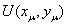be a vector then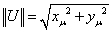. The dot product of vectors: Let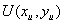andbe two vectors then the dot product of V and U is equal to: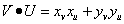. Norm of a vector and the cosine of a central angle: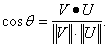For a central angle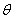we can rewrite the norm of a vector as relation with the product of the norms of vectors U and V and the product of vectors U and V, namely

Rapid Study Kit for "Title":
 Flash Movie Flash Game Flash Card Core Concept Tutorial Problem Solving Drill Review Cheat Sheet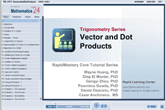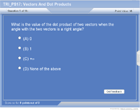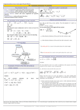"Title" Tutorial Summary : The study of vectors and dot products is mentioned in this tutorial. The properties of vectors are discussed in the examples and their use in application problems. The magnitude of a vector in comparison to the vector norm is mentioned in one of the definitions included in one of the example problems. Dot Products are discussed in this tutorial to assist on the introduction of their application in example problems. The use of a central and its cosine is discussed in one of the definition of a dot product and its norm.

 Tutorial Features: Specific Tutorial Features: • The properties of vectors and their graphical representation are presented in some examples. • Definition of a norm and its magnitude is included in one of the example problems. • Questions are included at the end of the tutorial to test knowledge and some of the concepts discussed in the tutorial. Series Features: • Concept map showing inter-connections of new concepts in this tutorial and those previously introduced. • Definition slides introduce terms as they are needed. • Visual representation of concepts • Animated examples—worked out step by step • A concise summary is given at the conclusion of the tutorial.

 "Title" Topic List: Definition of a vector Magnitude of a vector and its properties Addition of vectors and examples Opposite vectors and examples Vector dot product and examples Norm of a vector and its relation with the cosine of a central angle

See all 24 lessons in Trigonometry, including concept tutorials, problem drills and cheat sheets:
Teach Yourself Trigonometry Visually in 24 Hours